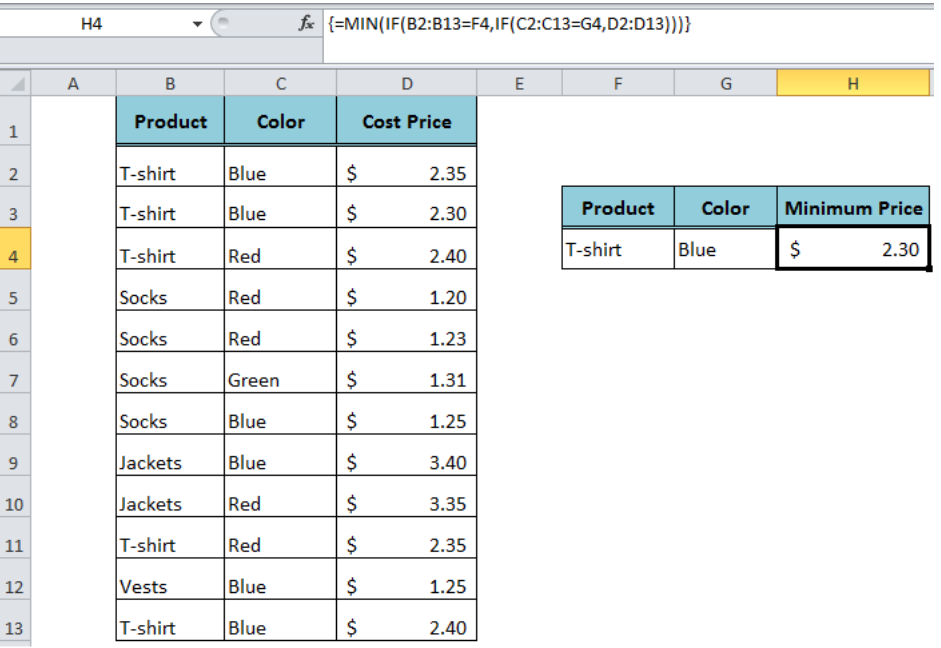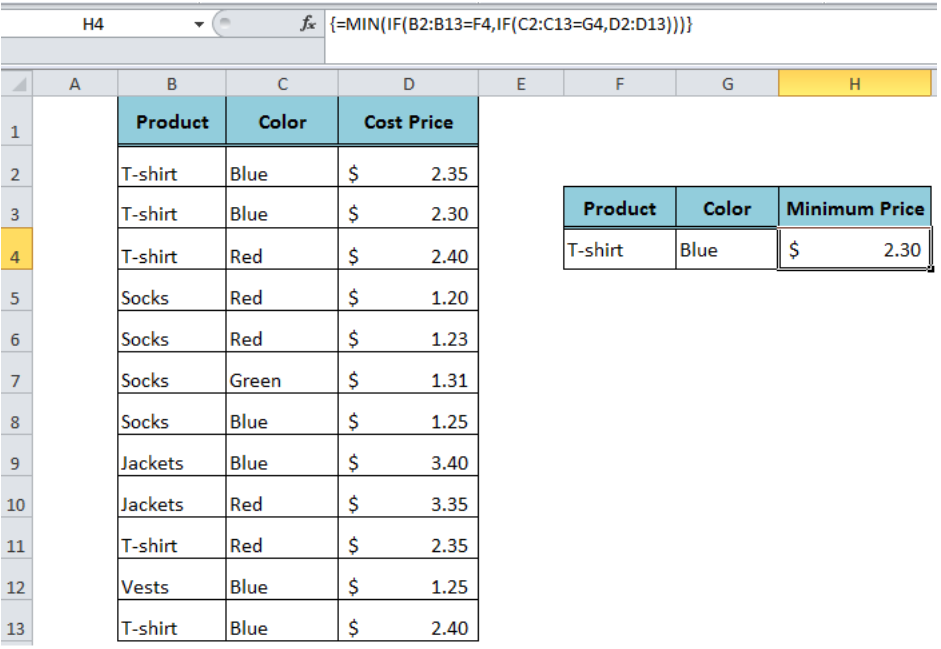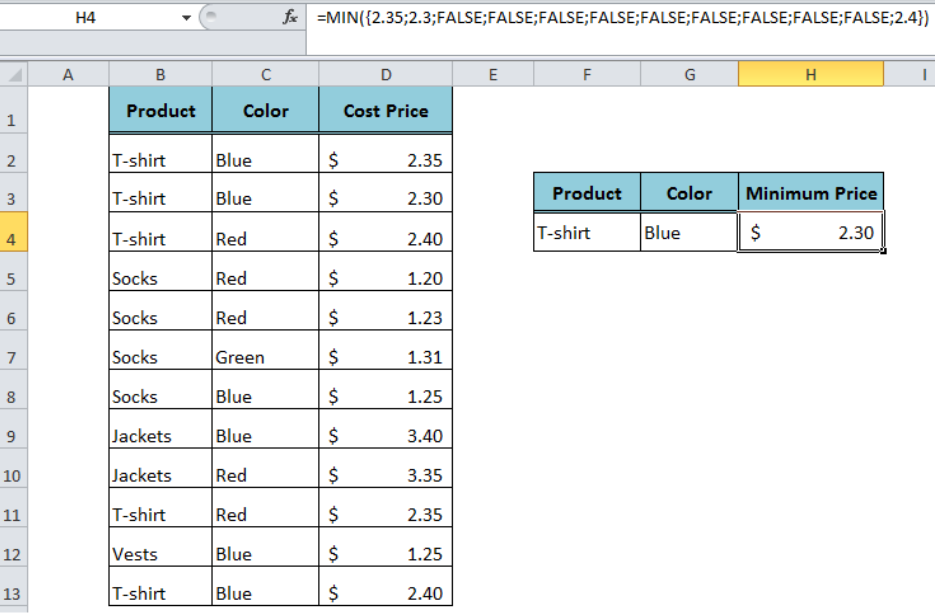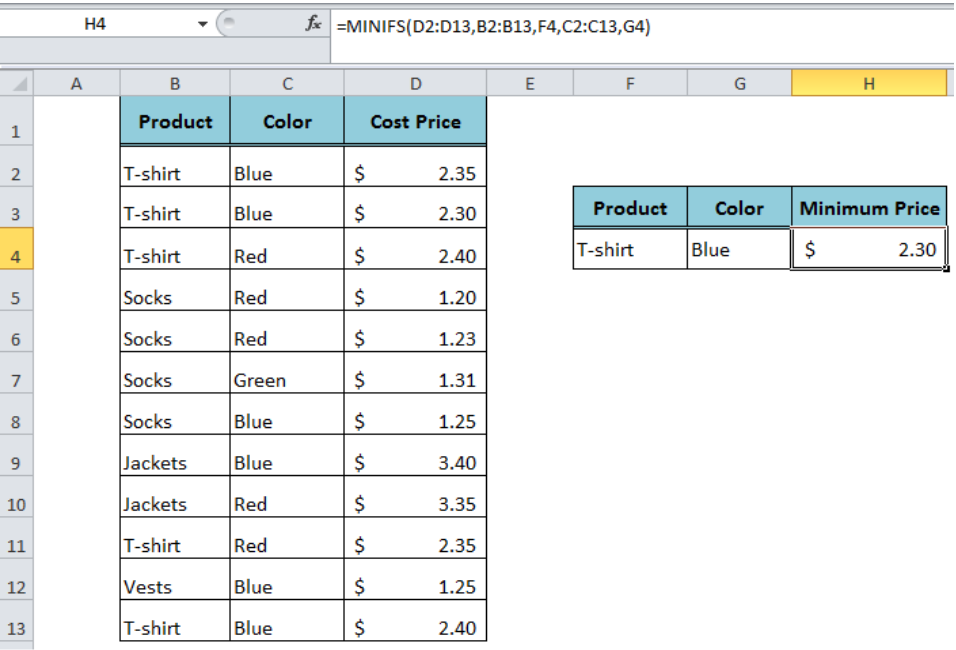Get instant live expert help with Excel or Google Sheets“My Excelchat expert helped me in less than 20 minutes, saving me what would have been 5 hours of work!”

#### Post your problem and you'll get expert help in seconds

Your message must be at least 40 characters
Our professional experts are available now. Your privacy is guaranteed.

# Minimum if multiple criteria

We use the MIN function to return the minimum value in a range of cells in Excel. But if we want to return the minimum or lowest value based on single criteria then we use the MIN function with IF function in an array formula because there is no built-in function in Excel. Furthermore, we can return minimum if multiple criteria using the MIN function with multiple IF functions in an array formula.Figure 1. Return Minimum Value If Multiple Criteria

## Using the MIN Function With Multiple IF Functions

In this method, we introduce nested IF statements to test multiple conditions inside the MIN function to return the minimum if multiple criteria. Suppose we want to estimate the minimum cost price for a specified product with a specified color from a data table. Products are listed in range B2: B13, with their colors in range C2: C13 and cost prices are listed in range D2: D13. We will apply the following formula in cell H4 as an array formula by pressing Ctrl+Shift+Enter keyboard keys;

`=MIN(IF(B2:B13=F4,IF(C2:C13=G4,D2:D13)))`Figure 2. Applying the MIN Function With Multiple IF Functions

Each IF statement returns an array of TRUE and FALSE logical values for each criteria value. When the range of values in “Value_If_True” argument is passed in the last IF statement, such as D2: D13. Then the final array returns the values from range D2: D13 where the previous arrays return TRUE in the corresponding position along with FALSE logical values, and the MIN function picks the lowest numeric value from this array.

`=MIN({2.35;2.3;FALSE;FALSE;FALSE;FALSE;FALSE;FALSE;FALSE;FALSE;FALSE;2.4})`Figure 3. Working of the MIN Function With Multiple IF Functions

## Using the MINIFS Function

The Excel MINIFS function has been introduced in Excel for Office 365 and later versions to return the minimum if multiple criteria without an array formula. Using this function, we can return the minimum value from a range of values based on one or more criteria in the following formula syntax;

`=MINIFS(range, criteria_range1, criteria1, [criteria_range2, criteria2], ...)`

The formula in our example would be;

`=MINIFS(D2:D13,B2:B13,F4,C2:C13,G4)`Figure 4. Using the MINIFS Function

## Instant Connection to an Expert through our Excelchat Service

Most of the time, the problem you will need to solve will be more complex than a simple application of a formula or function. If you want to save hours of research and frustration, try our live Excelchat service! Our Excel Experts are available 24/7 to answer any Excel question you may have. We guarantee a connection within 30 seconds and a customized solution within 20 minutes.

### Did this post not answer your question? Get a solution from connecting with the expert.Another blog reader asked this question today on Excelchat:## Subscribe to Excelchat.coAnother blog reader asked this question today on Excelchat: